Algebra

Equations

Inequalities

Graphs

Numbers

Calculus

Matrices

Tutorials

### Help: Examples of division

Here are some examples of typical expressions involving division. This table shows you what they would look like in a textbook and how they would need to be entered for QuickMath.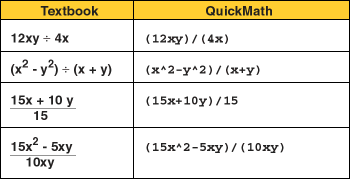# Division of Integers

From multiplication we have 4 x 6 = 24. When the number 6 is multiplied by 4, the result is 24. The number 6 is called the quotient of 24 divided by 4.

In notation, we write 24 : 4 = 6 or 24/4 = 6

The symbol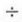, read "divided by," means division.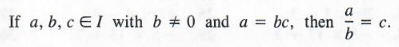When a/b = c, the number a is called the dividend, b is called the divisor, and c or a/b is called the quotient. The quotient a/b is also called a fraction; a is called the numerator and b is called the denominator of the fraction. Sometimes a and b are referred to as the terms of the fraction.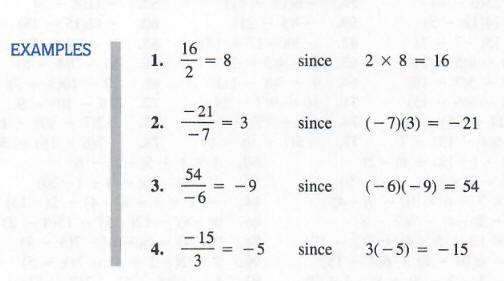Note The quotient of two positive or two negative numbers is a positive number. The quotient of a positive number divided by a negative number or a negative number divided by a positive number is a negative number.

Division by Zero

The product of zero and any number a  E I is zero.

Examples 0*5 =0,  0(-6) = 0

Division is defined from multiplication: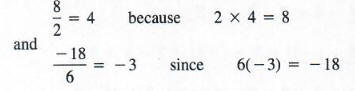Now, consider 4/0; we look for a number a E I such that 0 x a = 4.
There is no such number a, since 0 x a = 0 for all a E I.
Finally, consider 0/0; we look for a number b E I such that 0 x b = 0. This statement is true for any number b E I:

0*4 = 0
0*(-12) = 0
0x0 = 0

That is, b is not a unique number, and a quotient must be unique. Thus for any number a <> 0 we have:

0/a = 0

a/0 is not defined

0/0 is not a unique number, it is indeterminate

Note Since p/q is not defined when q = 0, all the denominators of the fractions will be assumed to be different from zero.

When an expression involves multiplications and divisions without grouping symbols, we perform the multiplications and divisions in the order in which they appear.

Examples: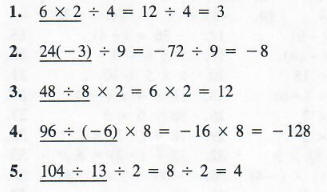When an expression involves the four arithmetic operations without grouping symbols, we perform multiplications and divisions in the order in which they appear before additions and subtractions.